# QlikView App Dev

Discussion Board for collaboration related to QlikView App Development.

Announcements
Welcome to Qlik Community! Check out our new navigation! FIND OUT MORE
cancel
Showing results for
Did you mean: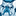Creator III

## How to combine multiple nested if Statements

Hello,

I have a table as shown below which rates Vendors as 'R' or 'Y' or 'G' based on a set of conditions for each of the 'Packages' present in the table: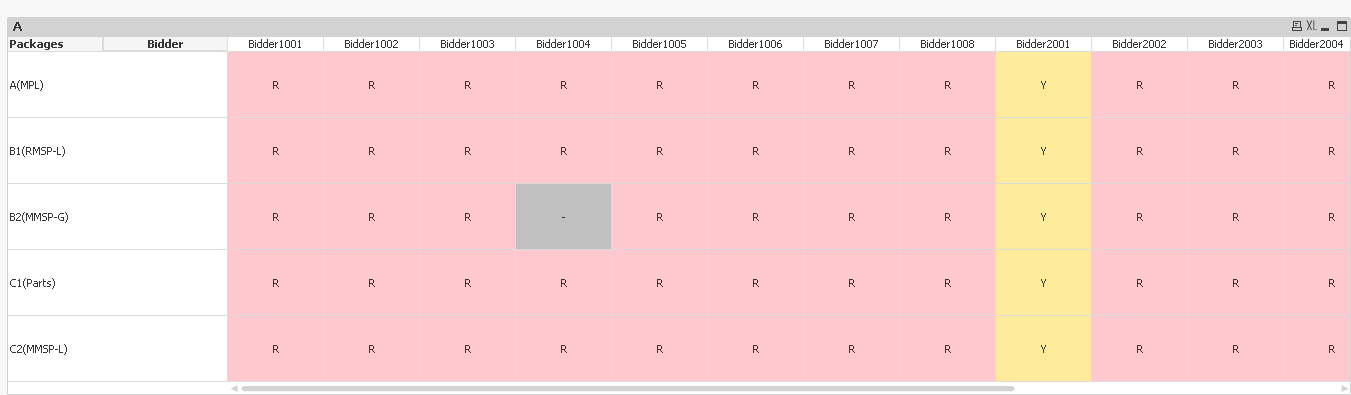The problem I am facing now is that for each package, the rating is based on consolidation of a set of qualifications which are captured in a nested in statement(for each one of the 5 Packages). I'm unable to figure out a way to combine these 5 nested if statements to display a final result in my table for each Vendor Package wise. The conditions(if statements) for each Package are specified below for your reference:

1.A(MPL):

if(Packages='A(MPL)' and Wildmatch(Bidder,'Bidder1***')=1 and (Count({<Appendix={'Appendix V'},No={'1A'},A={'COMPLY'}>}Items)=0) or (Count({<Appendix={'Appendix V'},={'COMPLY'}>}distinct No)<>Count({<Appendix={'Appendix V'},-={'N/A'}>}distinct No)) or (Package='Package A' and [HSE Evaluation Result]='R') or (Sum(If(VC.F22 ...

(Flag9='Fail' or Flag10='Fail' or Flag11='Fail' or Flag12='Fail' or Flag13='Fail' or Flag14='Fail') or (Flag15='Fail' or Flag16='Fail' or Flag17='Fail' or Flag18='Fail' or Flag19='Fail' or Flag20='Fail') or (Count({<Appendix={'Appendix V'},No={'14'},={'COMPLY'}>}distinct No)=0) or

(Package='Package A' and [Financial Evaluaton Result]='R'),'R',

if(Packages='A(MPL)' and Wildmatch(Bidder,'Bidder1***')=1 and (Count({<Appendix={'Appendix V'},No={'1A'},A={'COMPLY'}>}Items)=1) and (Count({<Appendix={'Appendix V'},={'COMPLY'}>}distinct No)=Count({<Appendix={'Appendix V'},-={'N/A'}>}distinct No)) and (Sum(If(VC.F22 = 'CV Submitted by Bidder', Responses0))>=4) and

(Count({<Appendix={'Appendix V'},No={'14'},={'COMPLY'}>}distinct No)=1) and (Package='Package A' and [HSE Evaluation Result]='Y') or (Flag12='Y...

'Y',

if(Packages='A(MPL)' and Wildmatch(Bidder,'Bidder2***')=1 and (Count({<Appendix={'Appendix V'},No={'1A'},A={'COMPLY'}>}Items)=0) or (Sum({<Appendix={'Appendix V(D) Time'}>})<60 and Sum({<Appendix={'Appendix V(D) Value'}>})<3000000) or (Count({<Appendix={'Appendix V'},={'COMPLY'}>}distinct No)<>Count({<Appendix={'Appendix V'},-={'N/A'}>}distinct No)) or (Package='Package A' and [HSE Evaluation Result]='R') or (Sum(If(VC.F22 ...

(Flag9='Fail' or Flag10='Fail' or Flag11='Fail' or Flag12='Fail' or Flag13='Fail' or Flag14='Fail') or (Flag15='Fail' or Flag16='Fail' or Flag17='Fail' or Flag18='Fail' or Flag19='Fail' or Flag20='Fail') or (Count({<Appendix={'Appendix V'},No={'14'},={'COMPLY'}>}distinct No)=0) or

(Package='Package A' and [Financial Evaluaton Result]='R'),'R',

if(Packages='A(MPL)' and Wildmatch(Bidder,'Bidder2***')=1 and (Count({<Appendix={'Appendix V'},No={'1A'},A={'COMPLY'}>}Items)=1) and (Sum({<Appendix={'Appendix V(D) Time'}>})>=60 or Sum({<Appendix={'Appendix V(D) Value'}>})>=3000000) and (Count({<Appendix={'Appendix V'},={'COMPLY'}>}distinct No)=Count({<Appendix={'Appendix V'},-={'N/A'}>}distinct No)) and (Sum(If(VC.F22 = 'CV Submitted by Bidder', Responses0))>=4) and

(Count({<Appendix={'Appendix V'},No={'14'},={'COMPLY'}>}distinct No)=1) and (Flag12='Yellow') or (Flag18='Yellow') or (Package='Package A' and ...

'Y', 'G'))))

2. B1(RMSP-L):

if(Packages='B1(RMSP-L)' and Wildmatch(Bidder,'Bidder1***')=1 and ((Count({<Appendix={'Appendix V(E) Time'},Sum1={">=60"}>}distinct [Equipment Category]) <> Count({<Appendix={'Appendix V(E) Time'},Sum1={">0"}>}DISTINCT([Equipment Category]))) or (Count({<Appendix={'Appendix V(E) Value'},Sum3={">=5000000"}>}distinct [Equipment Category]) <> Count({<Appendix={'Appendix V(E) Value'},Sum3={">0"}>}DISTINCT([Equipment Category])))) or (Count({<Appendix={'Appendix V'},[B(1)]={'COMPLY'}>}distinct No)<>Count({<Appendix={'Appendix V'},[B(1)]-={'N/A'}>}distinct No)) or (Count({<Appendix={'Appendix V'},No={'1B'},[B(1)]={'COMPLY'}>}Items)=0) or ([HSE Evaluation ResultB1]='R') or (Sum(If(VC.F22 = 'CV Submitted by Bidder', Responses0))<4) or ((Sum({<[Workshop Compliance]={'YES'}>}VJ.No))=3) or ((Sum({<[Workshop Compliance]={'YES'}>}VJ.No))=4) or (Count({<[Workshop Size (SQ Meter)]={">=400"},[Office Floor Area Size within Workshop (SQ Meter)]={">=100"},[Own/Lease Status]={'Owned','Leased','Own','Lease'}>}distinct([Workshop Name]))=0) or Flag36='Red' or

(Flag21='Fail' or Flag22='Fail' or Flag23='Fail' or Flag24='Fail' or Flag25='Fail' or Flag26='Fail' or Flag27='Fail' or Flag28='Fail') or (Flag29='Fail' or Flag30='Fail' or Flag31='Fail' or Flag32='Fail' or Flag33='Fail' or Flag34='Fail' or Flag35='Fail') or (Flag9='Fail' or Flag10='Fail' or Flag11='Fail' or Flag12='Fail' or Flag13='Fail' or Flag14='Fail') or (Flag15='Fail' or Flag16='Fail' or Flag17='Fail' or Flag18='Fail' or Flag19='Fail' or Flag20='Fail') or

(Count({<Appendix={'Appendix V'},No={'14'},[B(1)]={'COMPLY'}>}distinct No)=0) or [Financial Evaluation ResultB1]='R','R',

if(Packages='B1(RMSP-L)' and Wildmatch(Bidder,'Bidder1***')=1 and ((Count({<Appendix={'Appendix V(E) Time'},Sum1={">=60"}>}distinct [Equipment Category]) = Count({<Appendix={'Appendix V(E) Time'},Sum1={">0"}>}DISTINCT([Equipment Category]))) and (Count({<Appendix={'Appendix V(E) Value'},Sum3={">=5000000"}>}distinct [Equipment Category]) = Count({<Appendix={'Appendix V(E) Value'},Sum3={">0"}>}DISTINCT([Equipment Category])))) and (Count({<Appendix={'Appendix V'},[B(1)]={'COMPLY'}>}distinct No)=Count({<Appendix={'Appendix V'},[B(1)]-={'N/A'}>}distinct No)) and (Count({<Appendix={'Appendix V'},No={'1B'},[B(1)]={'COMPLY'}>}Items)=1) and (Sum(If(VC.F22 = 'CV Submitted by Bidder', Responses0))>=4) and (Count({<Appendix={'Appendix V'},No={'14'},[B(1)]={'COMPLY'}>}distinct No)=1) and ([HSE Evaluation ResultB1]='Y') or ((Sum({<[Workshop Compliance]={'YES'}>}VJ.No))=2) or Flag37='Yellow' or (Flag25='Yellow' and Flag26='Yellow') or Flag33='Yellow' or Flag12='Yellow' or Flag18='Yellow' or

[Financial Evaluation ResultB1]='Y','Y',

if(Packages='B1(RMSP-L)' and (Count({<Appendix={'Appendix V'},No={'1B'},[B(1)]={'COMPLY'}>}No)=0) or ((Count({<Appendix={'Appendix V(E) Time'},Sum1={">=60"}>}distinct [Equipment Category]) <> Count({<Appendix={'Appendix V(E) Time'},Sum1={">0"}>}DISTINCT([Equipment Category]))) or (Count({<Appendix={'Appendix V(E) Value'},Sum3={">=5000000"}>}distinct [Equipment Category]) <> Count({<Appendix={'Appendix V(E) Value'},Sum3={">0"}>}DISTINCT([Equipment Category])))) or (Count({<Appendix={'Appendix V'},[B(1)]={'COMPLY'}>}distinct No)<>Count({<Appendix={'Appendix V'},[B(1)]-={'N/A'}>}distinct No)) or ([HSE Evaluation ResultB1]='R') or (Sum(If(VC.F22 = 'CV Submitted by Bidder', Responses0))<4) or ((Sum({<[Workshop Compliance]={'YES'}>}VJ.No))=3) or ((Sum({<[Workshop Compliance]={'YES'}>}VJ.No))=4) or (Count({<[Workshop Size (SQ Meter)]={">=400"},[Office Floor Area Size within Workshop (SQ Meter)]={">=100"},[Own/Lease Status]={'Owned','Leased','Own','Lease'}>}distinct([Workshop Name]))=0) or Flag36='Red' or (Flag21='Fail' or Flag22='Fail' or Flag23='Fail' or Flag24='Fail' or Flag25='Fail' or Flag26='Fail' or Flag27='Fail' or Flag28='Fail') or

(Flag29='Fail' or Flag30='Fail' or Flag31='Fail' or Flag32='Fail' or Flag33='Fail' or Flag34='Fail' or Flag35='Fail') or (Flag9='Fail' or Flag10='Fail' or Flag11='Fail' or Flag12='Fail' or Flag13='Fail' or Flag14='Fail') or (Flag15='Fail' or Flag16='Fail' or Flag17='Fail' or Flag18='Fail' or Flag19='Fail' or Flag20='Fail') or (Count({<Appendix={'Appendix V'},No={'14'},[B(1)]={'COMPLY'}>}distinct No)=0) or

[Financial Evaluation ResultB1]='R','R',

if(Packages='B1(RMSP-L)' and (Count({<Appendix={'Appendix V'},No={'1B'},[B(1)]={'COMPLY'}>}No)=1) and ((Count({<Appendix={'Appendix V(E) Time'},Sum1={">=60"}>}distinct [Equipment Category]) = Count({<Appendix={'Appendix V(E) Time'},Sum1={">0"}>}DISTINCT([Equipment Category]))) and (Count({<Appendix={'Appendix V(E) Value'},Sum3={">=5000000"}>}distinct [Equipment Category]) = Count({<Appendix={'Appendix V(E) Value'},Sum3={">0"}>}DISTINCT([Equipment Category])))) and (Count({<Appendix={'Appendix V'},[B(1)]={'COMPLY'}>}distinct No)=Count({<Appendix={'Appendix V'},[B(1)]-={'N/A'}>}distinct No)) and (Sum(If(VC.F22 = 'CV Submitted by Bidder', Responses0))>=4) and (Count({<Appendix={'Appendix V'},No={'14'},[B(1)]={'COMPLY'}>}distinct No)=1) and ([HSE Evaluation ResultB1]='Y') or ((Sum({<[Workshop Compliance]={'YES'}>}VJ.No))=2) or Flag37='Yellow' or (Flag25='Yellow' and Flag26='Yellow') or

Flag33='Yellow' or Flag12='Yellow' or Flag18='Yellow' or [Financial Evaluation ResultB1]='Y','Y','G'))))

3. B2(MMSP-G):

if(Packages='B2(MMSP-G)' and (Count({<Appendix={'Appendix V'},[B(2)]={'Select'," '' "}>}distinct No)=Count({<Appendix={'Appendix V'},[B(2)]-={'N/A'}>}distinct No)),'-',

if(Packages='B2(MMSP-G)' and (Count({<Appendix={'Appendix V'},[B(2)]={'COMPLY'}>}distinct No)<>Count({<Appendix={'Appendix V'},[B(2)]-={'N/A'}>}distinct No)) or Count({<Appendix={'Appendix V'},No={'1B'},[B(2)]={'COMPLY'}>}Items)=0 or ([HSE Evaluation ResultB2]='R') or Sum(If(VC.F22 = 'CV Submitted by Bidder', Responses0))<4 or

((Count({<Appendix={'Appendix V(E) Time'},Sum2={">=60"}>}distinct [Equipment Category]) <> Count({<Appendix={'Appendix V(E) Time'},Sum2={">0"}>}DISTINCT([Equipment Category]))) or (Count({<Appendix={'Appendix V(E) Value'},Sum4={">=5000000"}>}distinct [Equipment Category]) <> Count({<Appendix={'Appendix V(E) Value'},Sum4={">0"}>}DISTINCT([Equipment Category])))) or

((Sum({<[Workshop Compliance]={'YES'}>}VJ.No))=3) or ((Sum({<[Workshop Compliance]={'YES'}>}VJ.No))=4) or (Count({<[Workshop Size (SQ Meter)]={">=400"},[Office Floor Area Size within Workshop (SQ Meter)]={">=100"},[Own/Lease Status]={'Owned','Leased','Own','Lease'}>}distinct([Workshop Name]))=0) or Flag36='Red' or (Flag21='Fail' or Flag22='Fail' or Flag23='Fail' or Flag24='Fail' or Flag25='Fail' or Flag26='Fail' or Flag27='Fail' or Flag28='Fail') or

(Flag29='Fail' or Flag30='Fail' or Flag31='Fail' or Flag32='Fail' or Flag33='Fail' or Flag34='Fail' or Flag35='Fail') or (Flag9='Fail' or Flag10='Fail' or Flag11='Fail' or Flag12='Fail' or Flag13='Fail' or Flag14='Fail') or (Flag15='Fail' or Flag16='Fail' or Flag17='Fail' or Flag18='Fail' or Flag19='Fail' or Flag20='Fail') or (Count({<Appendix={'Appendix V'},No={'14'},[B(2)]={'COMPLY'}>}distinct No)=0) or

[Financial Evaluation ResultB2]='R','R',

if(Packages='B2(MMSP-G)' and (Count({<Appendix={'Appendix V'},[B(2)]={'COMPLY'}>}distinct No)=Count({<Appendix={'Appendix V'},[B(2)]-={'N/A'}>}distinct No)) and (Count({<Appendix={'Appendix V'},No={'1B'},[B(2)]={'COMPLY'}>}Items)=1) and Sum(If(VC.F22 = 'CV Submitted by Bidder', Responses0))>=4 and ((Count({<Appendix={'Appendix V(E) Time'},Sum2={">=60"}>}distinct [Equipment Category]) = Count({<Appendix={'Appendix V(E) Time'},Sum2={">0"}>}DISTINCT([Equipment Category]))) and (Count({<Appendix={'Appendix V(E) Value'},Sum4={">=5000000"}>}distinct [Equipment Category]) = Count({<Appendix={'Appendix V(E) Value'},Sum4={">0"}>}DISTINCT([Equipment Category])))) and

((Sum({<[Workshop Compliance]={'YES'}>}VJ.No))=2) or Flag37='Yellow' or ([HSE Evaluation ResultB1]='Y') or (Flag25='Yellow' and Flag26='Yellow') or (Flag33='Yellow') or (Flag12='Yellow') or (Flag18='Yellow') or ([Financial Evaluation ResultB2]='Y'),'Y','G')))

4. C1(Parts):

if(Packages='C1(Parts)' and (Count({<Appendix={'Appendix V'},[C(1)]={'Select'," '' "}>}distinct No)=Count({<Appendix={'Appendix V'},[C(1)]-={'N/A'}>}distinct No)),'-',

if(Packages='C1(Parts)' and (Count({<Appendix={'Appendix V'},[C(1)]={'COMPLY'}>}distinct No)<>Count({<Appendix={'Appendix V'},[C(1)]-={'N/A'}>}distinct No)) or (Count({<Appendix={'Appendix V'},No={'1A'},[C(1)]={'COMPLY'}>}Items)=0) or ([HSE Evaluation ResultC1]='R') or

(Count({<Appendix={'Appendix V'},No={'14'},[C(1)]={'COMPLY'}>}distinct No)=0) or ([Financial Evaluation ResultC1]='R'),'R',

if(Packages='C1(Parts)' and (Count({<Appendix={'Appendix V'},[C(1)]={'COMPLY'}>}distinct No)=Count({<Appendix={'Appendix V'},[C(1)]-={'N/A'}>}distinct No)) and (Count({<Appendix={'Appendix V'},No={'1A'},[C(1)]={'COMPLY'}>}Items)=1) and (Count({<Appendix={'Appendix V'},No={'14'},[C(1)]={'COMPLY'}>}distinct No)=1) and

([HSE Evaluation ResultC1]='Y') or ([Financial Evaluation ResultC1]='Y'),'Y','G')))

5.C2(MMSP-L):

if(Packages='C2(MMSP-L)' and (Count({<Appendix={'Appendix V'},[C(2)]={'Select'," '' "}>}distinct No)=Count({<Appendix={'Appendix V'},[C(2)]-={'N/A'}>}distinct No)),'-',

if(Packages='C2(MMSP-L)' and (Count({<Appendix={'Appendix V'},[C(2)]={'COMPLY'}>}distinct No)<>Count({<Appendix={'Appendix V'},[C(2)]-={'N/A'}>}distinct No)) or (Count({<Appendix={'Appendix V'},No={'1B'},[C(2)]={'COMPLY'}>}Items)=0) or ([HSE Evaluation ResultC2]='R') or

(Count({<Appendix={'Appendix V'},No={'14'},[C(2)]={'COMPLY'}>}distinct No)=0) or ([Financial Evaluation ResultC2]='R') or ((Sum({<[Workshop Compliance]={'YES'}>}VJ.No))=3) or ((Sum({<[Workshop Compliance]={'YES'}>}VJ.No))=4) or (Count({<[Workshop Size (SQ Meter)]={">=400"},[Office Floor Area Size within Workshop (SQ Meter)]={">=100"},[Own/Lease Status]={'Owned','Leased','Own','Lease'}>}distinct([Workshop Name]))=0) or Flag36='Red','R',

if(Packages='C2(MMSP-L)' and (Count({<Appendix={'Appendix V'},[C(2)]={'COMPLY'}>}distinct No)=Count({<Appendix={'Appendix V'},[C(2)]-={'N/A'}>}distinct No)) and (Count({<Appendix={'Appendix V'},No={'1B'},[C(2)]={'COMPLY'}>}Items)=1) and (Count({<Appendix={'Appendix V'},No={'14'},[C(2)]={'COMPLY'}>}distinct No)=1) and

([HSE Evaluation ResultC2]='Y') or ([Financial Evaluation ResultC2]='Y') or ((Sum({<[Workshop Compliance]={'YES'}>}VJ.No))=2) or Flag37='Yellow','Y','G')))

I have tried combining all these 5 statements into one directly in the table one after the other but I'm not getting the correct result. What is the correct way of combining these statements? Thanks in advance!

1 Solution

Accepted SolutionsMVP

Try Pick(Match(....))

7 RepliesMVP

What is the output you expect to see? I am not sure how easy or difficult it would be help you resolve your issue, but let's start with the expected outputCreator III
Author

Hi Sunny! The output I want to see is a summary of results of 5 tables actually:

1. A(MPL):2.B1(RMSP-L):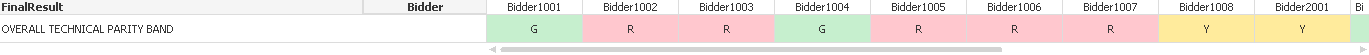3.B2(MMSP-G):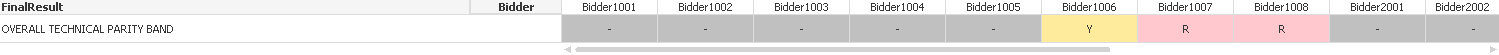4. C1(Parts):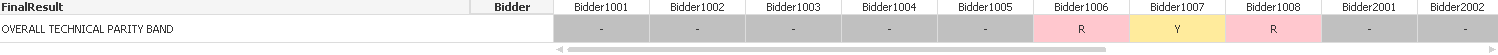5. C2(MMSP-L):The expressions used for these individual tables are available in the original post. Now the final result I want is a summary table with Package as one column and Bidders as the other columns with the responses from above tables shown in the final table. Let me know if something is not clear! Thanks!MVP

These 5 tables are not in the attached sample, right? Would you be able to add them and re-post the sample?Creator III
Author

Here it is Sunny. I have added the tables in a new sheet named 'Tables'. Thanks!MVP

Try Pick(Match(....))Creator III
Author

Wow. That was simple. If I understood the function correctly, it just picks one of the packages from the list one by one and matches it to the expressions given below in order and thus they are displayed in table based on this picking and matching. Seems like a really really powerful function. Is that correct Sunny? Thanks a lot for your help!MVP

Yup, you are absolutely right. It is a very efficient way of doing if statements. Not only does it make the syntax look nicer, but it also gives better performance than if statements.